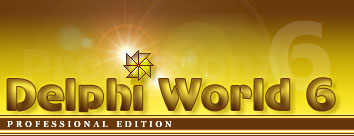Как получить имя программы, с которой ассоциировано то или иное расширение

 Автор: Олег Кулабухов

 Юзер подходит к программисту и спрашивает: - У меня почемуто пейджмейкеровский файл в ворде не открывается. - И не откроется, там же расширения разные. - Так я его переименовал.

 ```uses {\$IFDEF WIN32} Registry; {We will get it from the registry} {\$ELSE} IniFiles; {We will get it from the win.ini file} {\$ENDIF} {\$IFNDEF WIN32} const MAX_PATH = 144; {\$ENDIF} function GetProgramAssociation(Ext: string): string; var {\$IFDEF WIN32} reg: TRegistry; s: string; {\$ELSE} WinIni: TIniFile; WinIniFileName: array[0..MAX_PATH] of char; s: string; {\$ENDIF} begin {\$IFDEF WIN32} s := ''; reg := TRegistry.Create; reg.RootKey := HKEY_CLASSES_ROOT; if reg.OpenKey('.' + ext + '\shell\open\command', false) <> false then begin {The open command has been found} s := reg.ReadString(''); reg.CloseKey; end else begin {perhaps thier is a system file pointer} if reg.OpenKey('.' + ext, false) <> false then begin s := reg.ReadString(''); reg.CloseKey; if s <> '' then begin {A system file pointer was found} if reg.OpenKey(s + '\shell\open\command', false) <> false then {The open command has been found} s := reg.ReadString(''); reg.CloseKey; end; end; end; {Delete any command line, quotes and spaces} if Pos('%', s) > 0 then Delete(s, Pos('%', s), length(s)); if ((length(s) > 0) and (s = '"')) then Delete(s, 1, 1); if ((length(s) > 0) and (s[length(s)] = '"')) then Delete(s, Length(s), 1); while ((length(s) > 0) and ((s[length(s)] = #32) or (s[length(s)] = '"'))) do Delete(s, Length(s), 1); {\$ELSE} GetWindowsDirectory(WinIniFileName, sizeof(WinIniFileName)); StrCat(WinIniFileName, '\win.ini'); WinIni := TIniFile.Create(WinIniFileName); s := WinIni.ReadString('Extensions', ext, ''); WinIni.Free; {Delete any command line} if Pos(' ^', s) > 0 then Delete(s, Pos(' ^', s), length(s)); {\$ENDIF} result := s; end; procedure TForm1.Button1Click(Sender: TObject); begin ShowMessage(GetProgramAssociation('gif')); end; ```

Проект Delphi World © Выпуск 2002 - 2020
Автор проекта: Разработка программного обеспечения
Купить франшизу этого проекта можно здесь.